### IMO Shortlist 1996 problem C7

Kvaliteta:
Avg: 0,0
Težina:
Avg: 9,0
let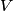$V$ be a finitive set and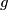$g$ and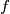$f$ be two injective surjective functions from$V$to$V$.let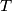$T$ and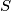$S$ be two sets such that they are defined as following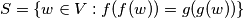$S = \{w \in V: f(f(w)) = g(g(w))\}$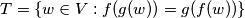$T = \{w \in V: f(g(w)) = g(f(w))\}$
we know that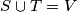$S \cup T = V$, prove:
for each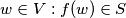$w \in V : f(w) \in S$ if and only if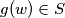$g(w) \in S$
Izvor: Međunarodna matematička olimpijada, shortlist 1996